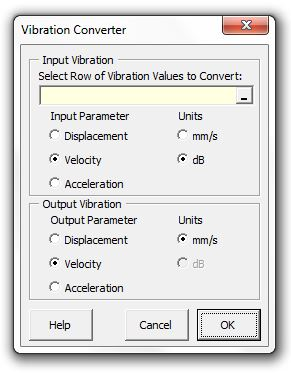### Strutt Help

Vibration Converter    1/1, 1/3

Strutt|Vibration|Vibration Converter - Converts a row of displacement, velocity or acceleration data into the equivalent displacement, velocity or acceleration, in either engineering units or dB. Highlight the row of values to be converted and select the relvant parameter and what it is to be converted to, and the result will be inserted into the active row in the worksheet.Strutt implements the following formulae to make the conversion:

• Convert displacement (d) to decibels:

dB_d = 20log_10(d/d_(ref)) | d_(ref) = 10^-12 \ m

• Convert velocity (v) to decibels:

dB_v = 20log_10(v/v_(ref)) | v_(ref) = 10^-9 \ m//s

• Convert acceleration (a) to decibels:

dB_a = 20log_10(a/a_(ref)) | a_(ref) = 10^-6 \ m//s^2

Velocity, acceleration and displacement are related by the following formula:

|v| = 2 pi f * d

|a| = (2 pi f)^2 * d = 2 pi f * v

Using the above formulae, a vibration level expressed as either velocity, acceleration or displacement, in either engineering units or decibels, may be converted to an equivalent expression.